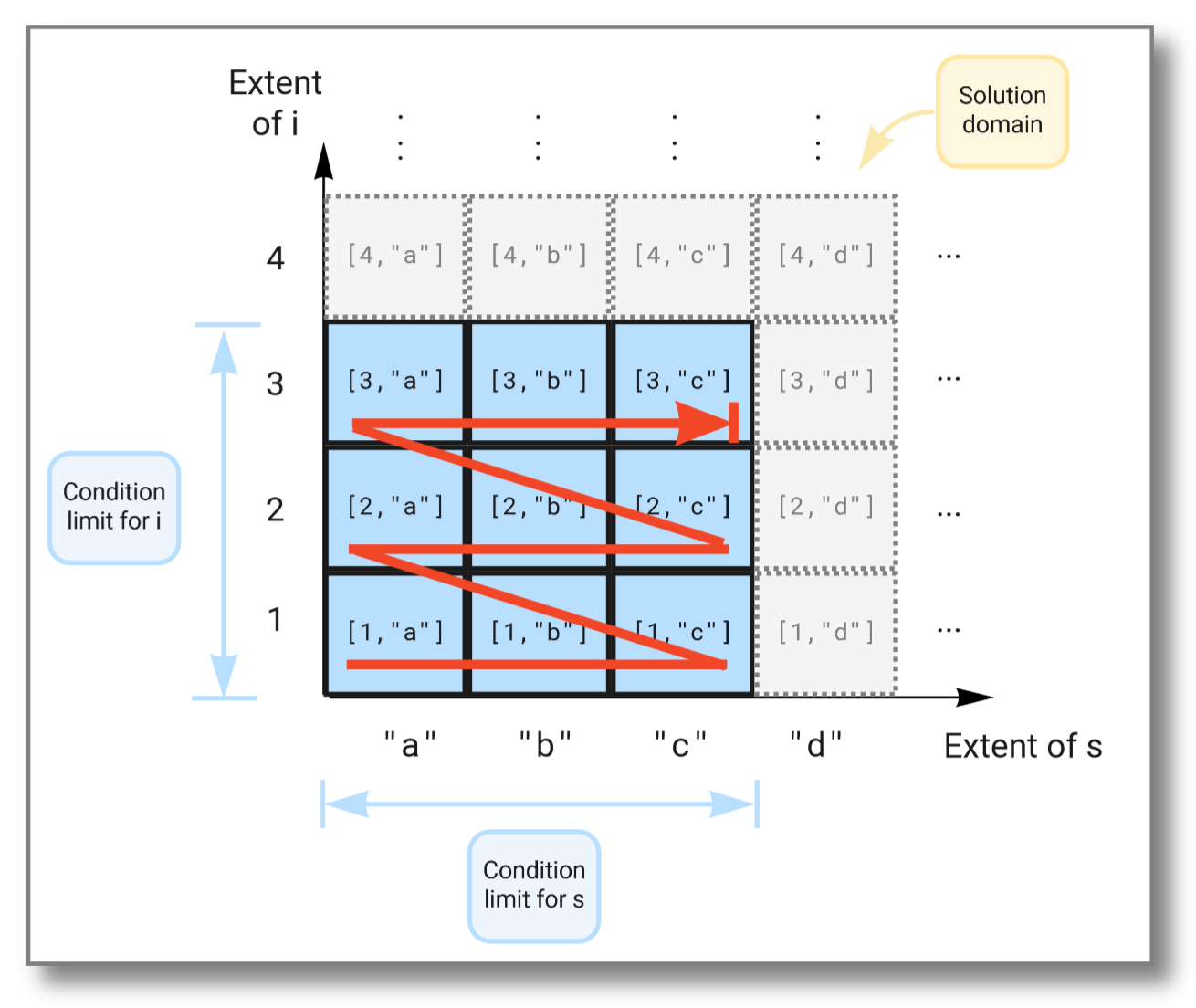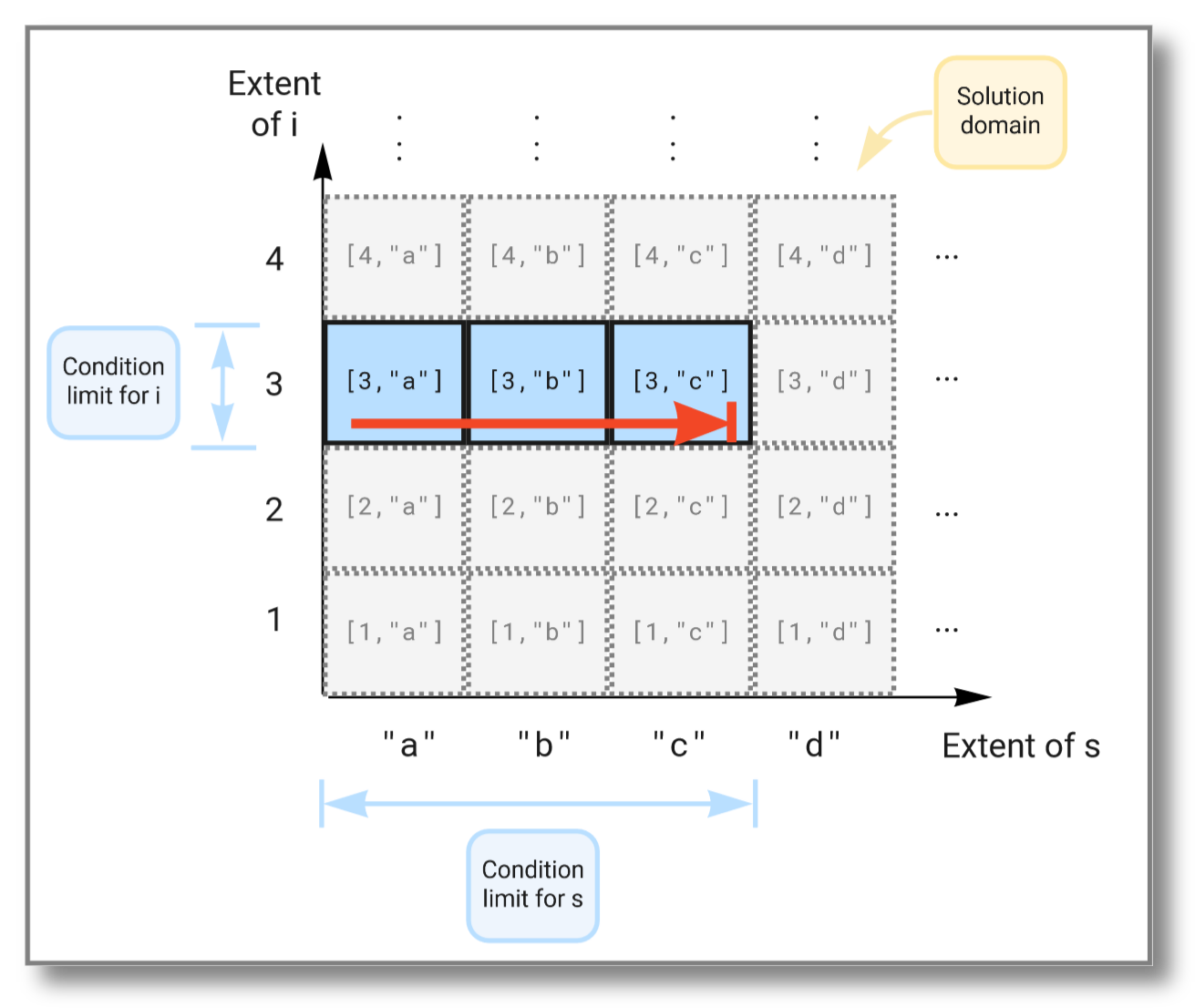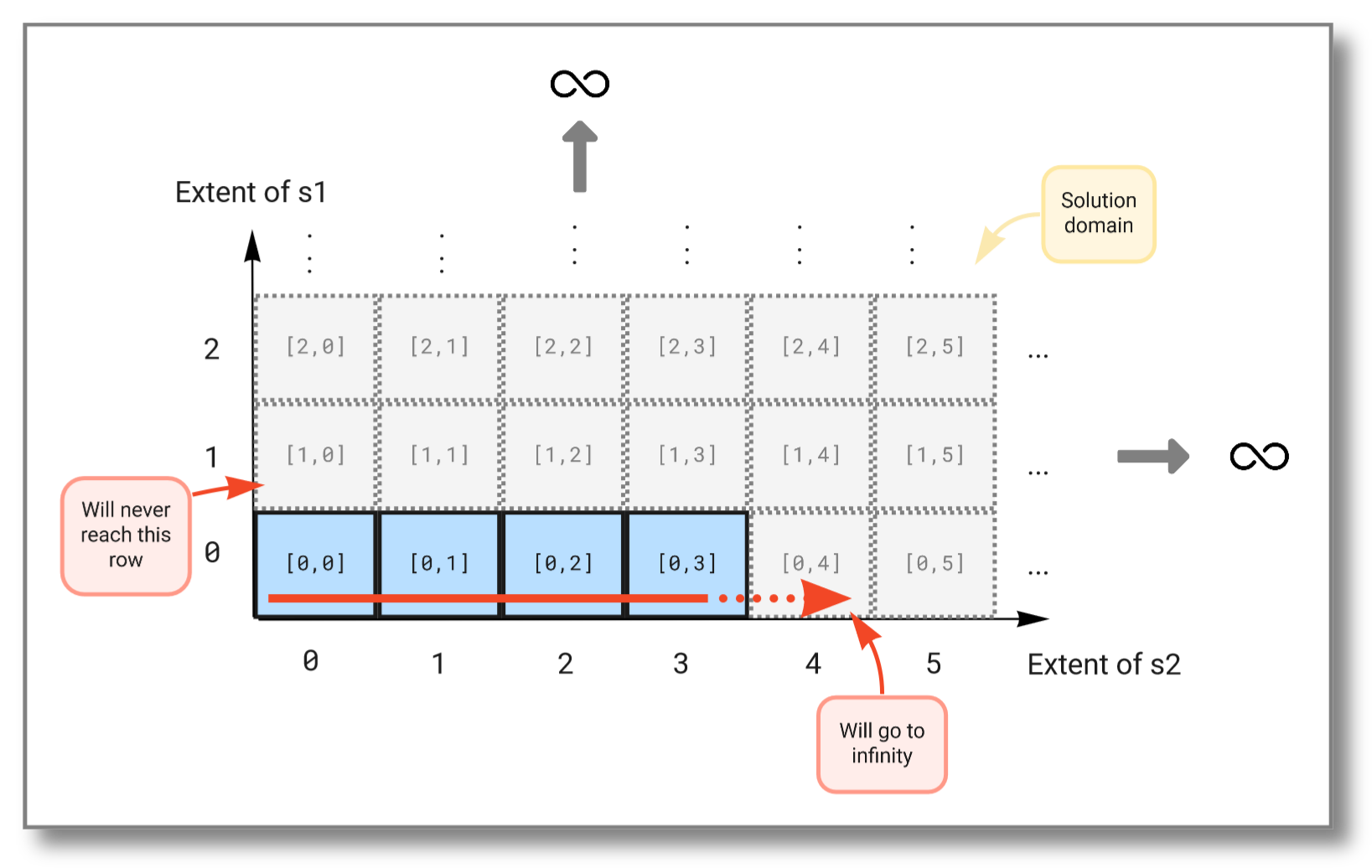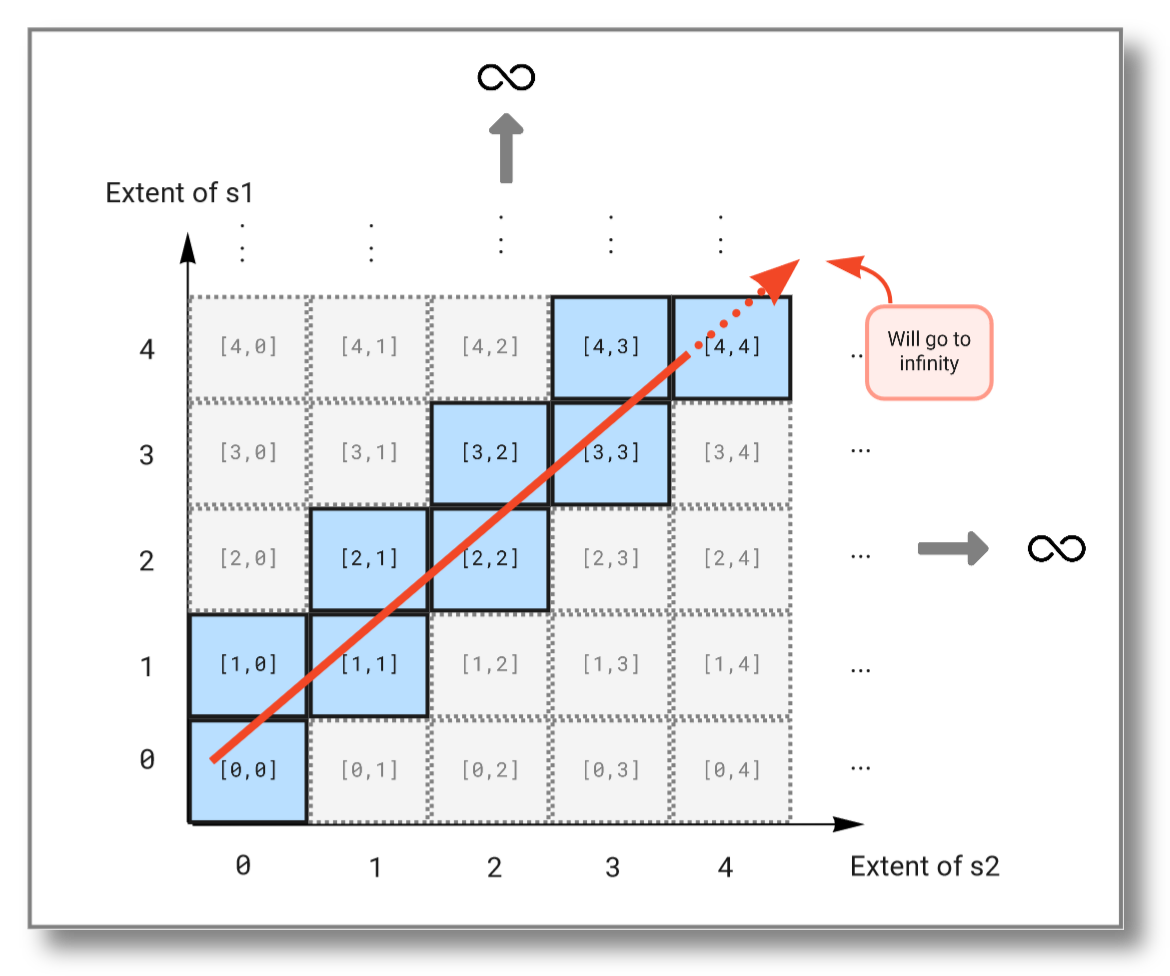# Queries

Queries retrieve objects having specified properties from the database. A query can be one of the following:

It can be a function call to a built-in or user defined OSQL function returning objects based on the function's arguments.

It can be a set query to search the database for a set of objects having properties fulfilling a query condition specified as a logical predicate.

It can be a vector query to construct an ordered sequence (vector) of objects fulfilling a query condition.

It can be a Stream query to extract a possibly infinite stream of objects from one or several other streams of objects.

## Type extent and the solution domain​

Every type t has an extent(t) being the set of all objects of type t. For example, the extent of the type Integer is "all integers from negative infinity to positive infinity", or $\{-\infty, ..., -2, -1, 0, 1, 2, ..., \infty\}$.

A select statement forms the Cartesian product of the extents of all variables in the from clause. The solution domain is the span of all extents. The where clause then specifies conditions limiting the emitted result to a subset of the solution domain.

For example, consider the select statement below. It forms a Cartesian product of the extent of i, which is the set of all possible integers, with the extent of s, which is the set of all possible strings. The where clause has two conditions. One that limits i to the set $\{1,2,3\}$, and one that limits s to the set $\{"a","b","c"\}$. This restricts the result to the Cartesian product of $\{1,2,3\}$ and $\{"a","b","c"\}$.

select i, s  from Integer i, Charstring s where i in bag(1,2,3)   and s in bag("a","b","c");

Running the above query gives the following result:

[1,"a"][1,"b"][1,"c"][2,"a"][2,"b"][2,"c"][3,"a"][3,"b"][3,"c"]

The figure below illustrates how the extents span the solution domain, and how the result is produced as the Cartesian product of the two extents limited by the conditions in the where clause:We can limit the solution further by imposing additional conditions in the where clause, like this:

select i, s  from Integer i, Charstring s where i in bag(1,2,3)   and s in bag("a","b","c")   and i > 2;

Running the above query gives the following result:

[3,"a"][3,"b"][3,"c"]

This time the subset of the solution space is further limited due to the extra condition on i and now looks like this:For a Stream query, let's consider binding variables to infinite subsets in the solution domain. We can do this by using streams that never end. For example, heartbeat() generates a stream of seconds emitted at a given pace. This stream is infinite since time has no end. The select statement below tries to combine two such streams, but as we will see, taking the Cartesian product between two infinite sets will not work. Try running the query:

select s1, s2  from Number s1, Number s2 where s1 in heartbeat(1)   and s2 in heartbeat(1);

The result should be:

[0,0][0,1][0,2][0,3]...

We see that the first stream never increases because the second stream (which is evaluated first) never finishes. The figure below illustrates how the Cartesian product "travels" through the solution domain:To combine infinite streams you must instead use the pivot() function. It combines the outputs of multiple streams into one vector and outputs a new vector each time one of the streams emits a result. The query below combines the streams from the previous example into a single stream:

select v  from Stream of Number s1, Stream of Number s2, Vector v where s1 = heartbeat(1)   and s2 = heartbeat(1)   and v in pivot([s1,s2]);

The result should be:

[0,null][0,0][1,0][1,1][2,1][2,2][3,2][3,3][4,3][4,4]...

As you see, this query will continue to infinity but include the values from both streams. If we look at the subset emitted from the solution domain, we see that the query "travels" diagonally through the solution domain: